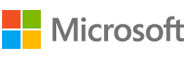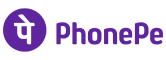New update is available. Click here to update.
Topics

# Merge K Sorted Arrays

Moderate0/80
Average time to solve is 15m+18 more companies

## Problem statement

You have been given ‘K’ different arrays/lists, which are sorted individually (in ascending order). You need to merge all the given arrays/list such that the output array/list should be sorted in ascending order.

Detailed explanation ( Input/output format, Notes, Images )
Constraints :
``````1 <= T <= 5
1 <= K <= 5
1 <= N <= 20
-10^5 <= DATA <= 10^5

Time Limit: 1 sec
``````
Sample Input 1:
``````1
2
3
3 5 9
4
1 2 3 8
``````
Sample Output 1:
``````1 2 3 3 5 8 9
``````
Explanation of Sample Input 1:
``````After merging the two given arrays/lists [3, 5, 9] and [ 1, 2, 3, 8], the output sorted array will be [1, 2, 3, 3, 5, 8, 9].
``````
Sample Input 2:
``````1
4
3
1 5 9
2
45 90
5
2 6 78 100 234
1
0
``````
Sample Output 2:
``````0 1 2 5 6 9 45 78 90 100 234
``````
Explanation of Sample Input 2 :
``````After merging the given arrays/lists [1, 5, 9], [45, 90], [2, 6, 78, 100, 234] and , the output sorted array will be [0, 1, 2, 5, 6, 9, 45, 78, 90, 100, 234].
``````Console